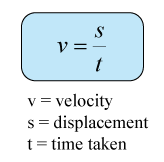# Finding Velocity from Ticker Tape

Finding Velocity
The velocity of motion can be determined by using ticker tape through the following equation:Caution!:
t is the time taken from the first dot to the last dot of the distance measured.

Example:The diagram above shows a strip of ticker tape that was pulled through a ticker tape timer that vibrated at 50 times a second. What is the

1. time taken from the first dot to the last dot?
2. average velocity of the object that is represented by the ticker tape?